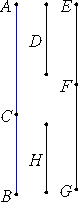# Proposition 52

To find the fifth binomial line.

Set out two numbers AC and CB such that AB does not have to either of them the ratio which a square number has to a square number. Set out any rational straight line D, and let EF be commensurable with D. Then EF is rational.X.6,Cor.

Let it be contrived that CA is to AB as the square on EF is to the square on FG.

But CA does not have to AB the ratio which a square number has to a square number, therefore neither does the square on EF have to the square on FG the ratio which a square number has to a square number.

Therefore EF and FG are rational straight lines commensurable in square only, therefore EG is binomial.

I say next that it is also a fifth binomial straight line.

V.7.Cor

Since CA is to AB as the square on EF is to the square on FG, therefore, inversely, BA is to AC as the square on FG is to the square on FE. Therefore the square on GF is greater than the square on FE.

V.19,Cor.

Let then the sum of the squares on EF and H equal the square on GF. Then, in conversion, the number AB is to BC as the square on GF is to the square on H.

But AB does not have to BC the ratio which a square number has to a square number, therefore neither does the square on FG have to the square on H the ratio which a square number has to a square number.

X.9

Therefore FG is incommensurable in length with H, so that the square on FG is greater than the square on FE by the square on a straight line incommensurable with FG.

And GF and FE are rational straight lines commensurable in square only, and the lesser term EF is commensurable in length with the rational straight line D set out.

Therefore EG is a fifth binomial straight line.

Q.E.D.

## Guide

This proposition is not used in the rest of the Elements.